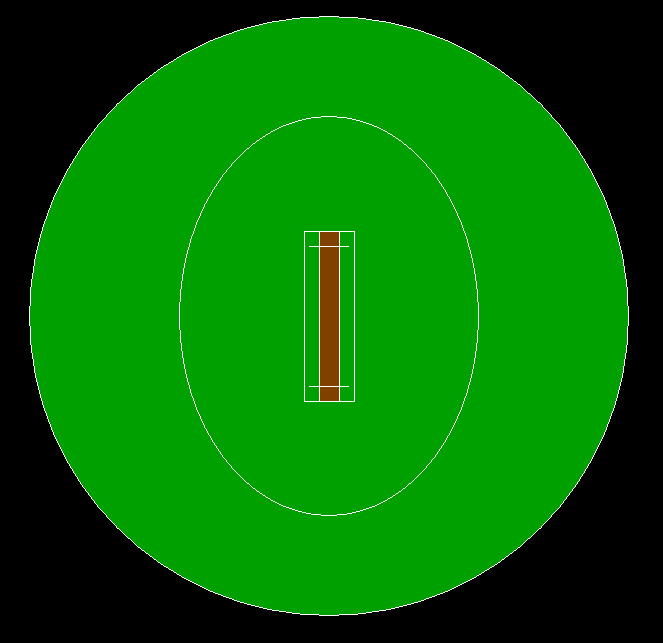# C program to draw a cricket ground using computer graphics

• Difficulty Level : Expert
• Last Updated : 04 May, 2021

In this article, we will discuss how to draw a 2D cricket ground is being designed using computer graphics.

Approach:

Below is the program for the above approach:

## C

 `// C program for the above approach ` ` `  `#include ` `#include ` `#include ` ` `  `// Driver Code ` `void` `main() ` `{ ` `    ``int` `gd = DETECT, gm; ` ` `  `    ``// Initialize of gdriver with ` `    ``// DETECT macros ` `    ``initgraph(&gd, &gm, ``"C:\\turboc3\\bgi"``); ` ` `  `    ``// Ground Outline ` `    ``circle(700, 350, 300); ` ` `  `    ``// Coloring Green ` `    ``setfillstyle(SOLID_FILL, GREEN); ` `    ``floodfill(402, 350, 15); ` ` `  `    ``// 30 Yards Outline ` `    ``ellipse(700, 350, 0, 360, 150, 200); ` ` `  `    ``// Pitch Outer Line ` `    ``rectangle(675, 265, 725, 435); ` ` `  `    ``// Pitch Inner Line ` `    ``rectangle(690, 265, 710, 435); ` ` `  `    ``// Coloring Pitch Brown ` `    ``setfillstyle(SOLID_FILL, BROWN); ` `    ``floodfill(695, 300, 15); ` ` `  `    ``// Upper Stump Line ` `    ``rectangle(690, 265, 710, 280); ` `    ``line(680, 280, 720, 280); ` ` `  `    ``// Lower Stump Line ` `    ``rectangle(690, 435, 710, 420); ` `    ``line(680, 420, 720, 420); ` ` `  `    ``// Hold Screen For A While ` `    ``getch(); ` ` `  `    ``// Close the initialized gdriver ` `    ``closegraph(); ` `} `

Output:My Personal Notes arrow_drop_up
Recommended Articles
Page :